# How to Calculate and Solve for Current Density | Corrosion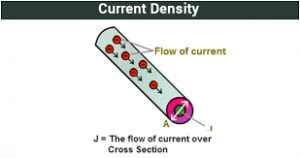The image above represents current density.

To compute for current density, three essential parameters are needed and these parameters are Number of Electron Charge Present (z), Force (F) and Rate of Heterogeneous Reaction (V).

The formula for calculating current density:

j = zFV

Where:

j = Current Density
z = Number of Electron Charge Present
F = Force
V = Rate of Heterogeneous Reaction

Let’s solve an example;
Find the current density when the number of electron charge present is 6, the force is 4 and the rate of heterogeneous reaction is 8.

This implies that;

z = Number of Electron Charge Present = 6
F = Force = 4
V = Rate of Heterogeneous Reaction = 8

j = zFV
j = (6) (4) (8)
j = 192

Therefore, the current density is 192 A/m².

Calculating the Number of Electron Charge Present when the Current Density, the Force and the Rate of Heterogeneous Reaction is Given.

z = j / FV

Where;

z = Number of Electron Charge Present
j = Current Density
F = Force
V = Rate of Heterogeneous Reaction

Let’s solve an example;
Find the number of electron charge present when the current density is 40, the force is 2 and the rate of heterogeneous reaction is 4.

This implies that;

j = Current Density = 40
F = Force = 2
V = Rate of Heterogeneous Reaction = 4

z = j / FV
z = 40 / 2 x 4
z = 40 / 8
z = 5

Therefore, the number of electron charge present is 5.

Calculating the Force when the Current Density, the Number of Electron Charge Present and the Rate of Heterogeneous Reaction is Given.

F = j / zV

Where;

F = Force
j = Current Density
z = Number of Electron Charge Present
V = Rate of Heterogeneous Reaction

Let’s solve an example;
Find the force when the current density is 30, the number of electron charge present is 8 and the rate of heterogeneous reaction is 2.

This implies that;

j = Current Density = 30
z = Number of Electron Charge Present = 8
V = Rate of Heterogeneous Reaction = 2

F = j / zV
F = 30 / 2 x 8
F = 30 / 16
F = 1.88

Therefore, the force is 1.88 N.

Calculating the Rate of Heterogeneous Reaction when the Current Density, the Number of Electron Charge Present and the Force is Given.

V = j / zF

Where;

V = Rate of Heterogeneous Reaction
j = Current Density
z = Number of Electron Charge Present
F = Force

Let’s solve an example;
Find the rate of heterogeneous reaction when the current density is 24, the number of electron charge present is 3 and the force is 4.

This implies that;

j = Current Density = 24
z = Number of Electron Charge Present = 3
F = Force = 4

V = j / zF
V = 24 / 3 x 4
V = 24 / 12
V = 2

Therefore, the rate of heterogeneous reaction is 2.

Nickzom Calculator – The Calculator Encyclopedia is capable of calculating the current density.

To get the answer and workings of the current density using the Nickzom Calculator – The Calculator Encyclopedia. First, you need to obtain the app.

You can get this app via any of these means:

To get access to the professional version via web, you need to register and subscribe for NGN 2,000 per annum to have utter access to all functionalities.
You can also try the demo version via https://www.nickzom.org/calculator

Apple (Paid) – https://itunes.apple.com/us/app/nickzom-calculator/id1331162702?mt=8
Once, you have obtained the calculator encyclopedia app, proceed to the Calculator Map, then click on Materials and Metallurgical under Engineering.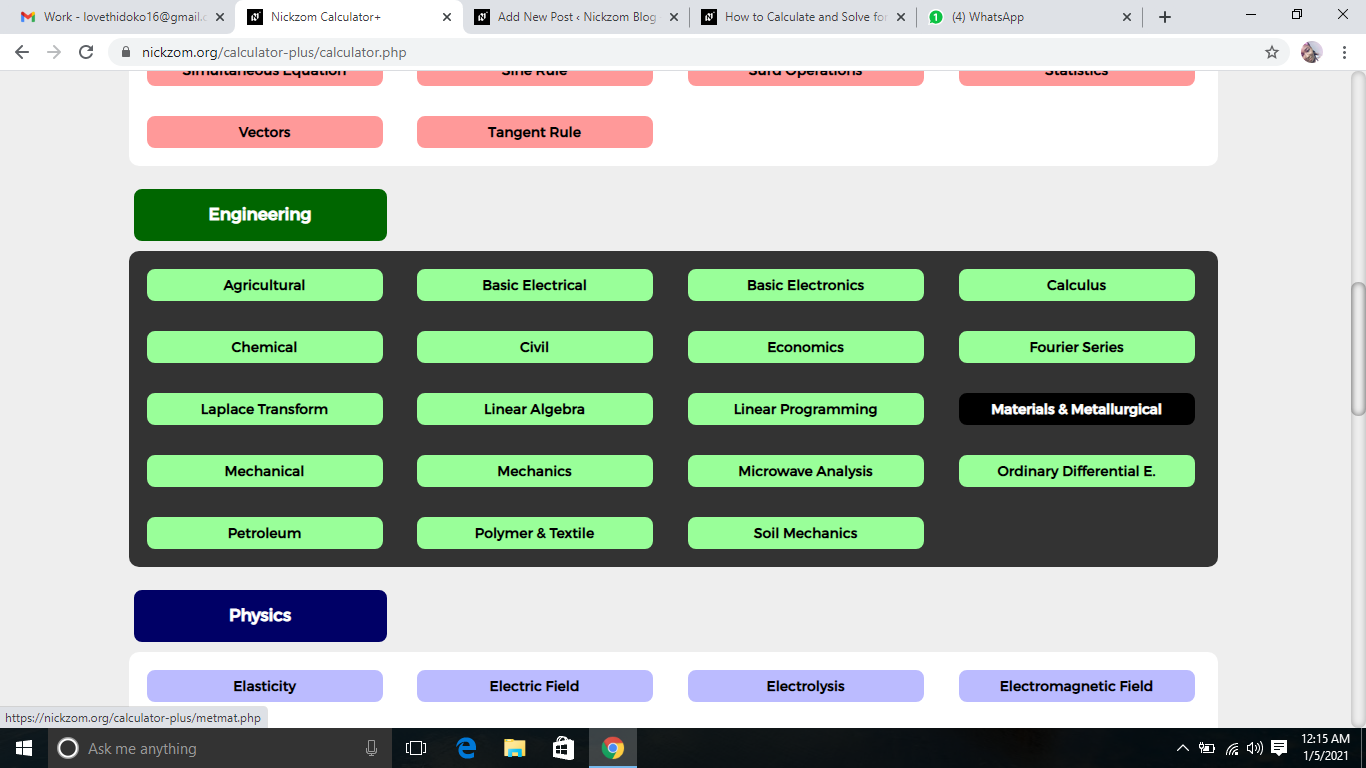Now, Click on Corrosion under Materials and Metallurgical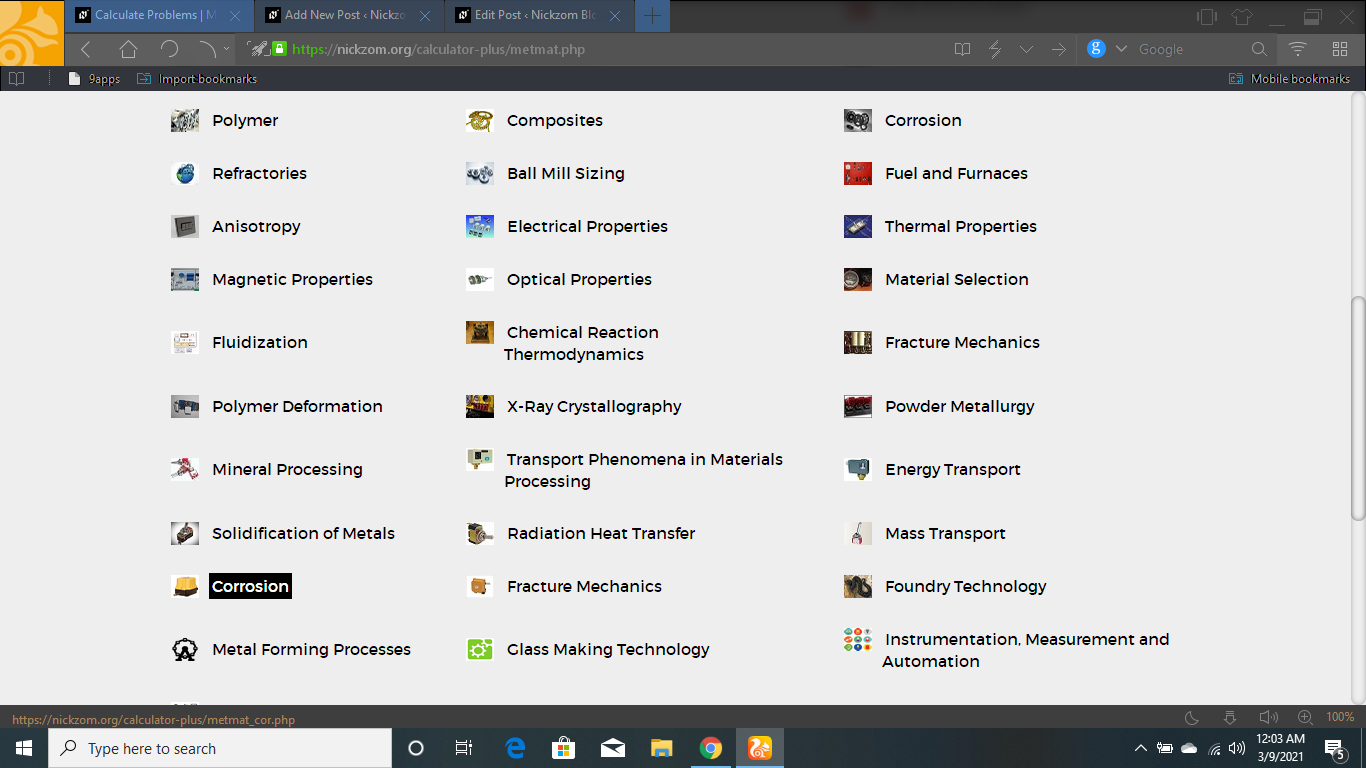Now, Click on Current Density under Corrosion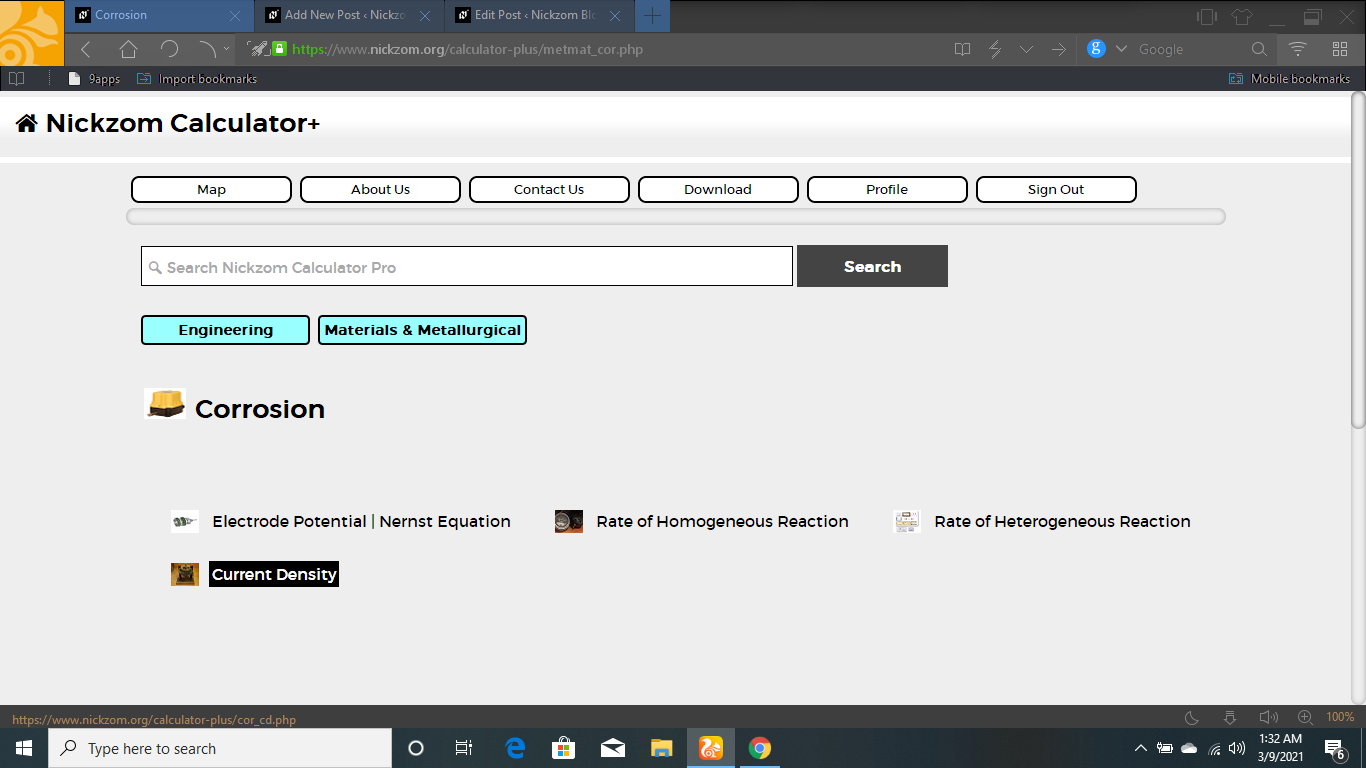The screenshot below displays the page or activity to enter your values, to get the answer for the current density according to the respective parameters which is the Number of Electron Charge Present (z), Force (F) and Rate of Heterogeneous Reaction (V).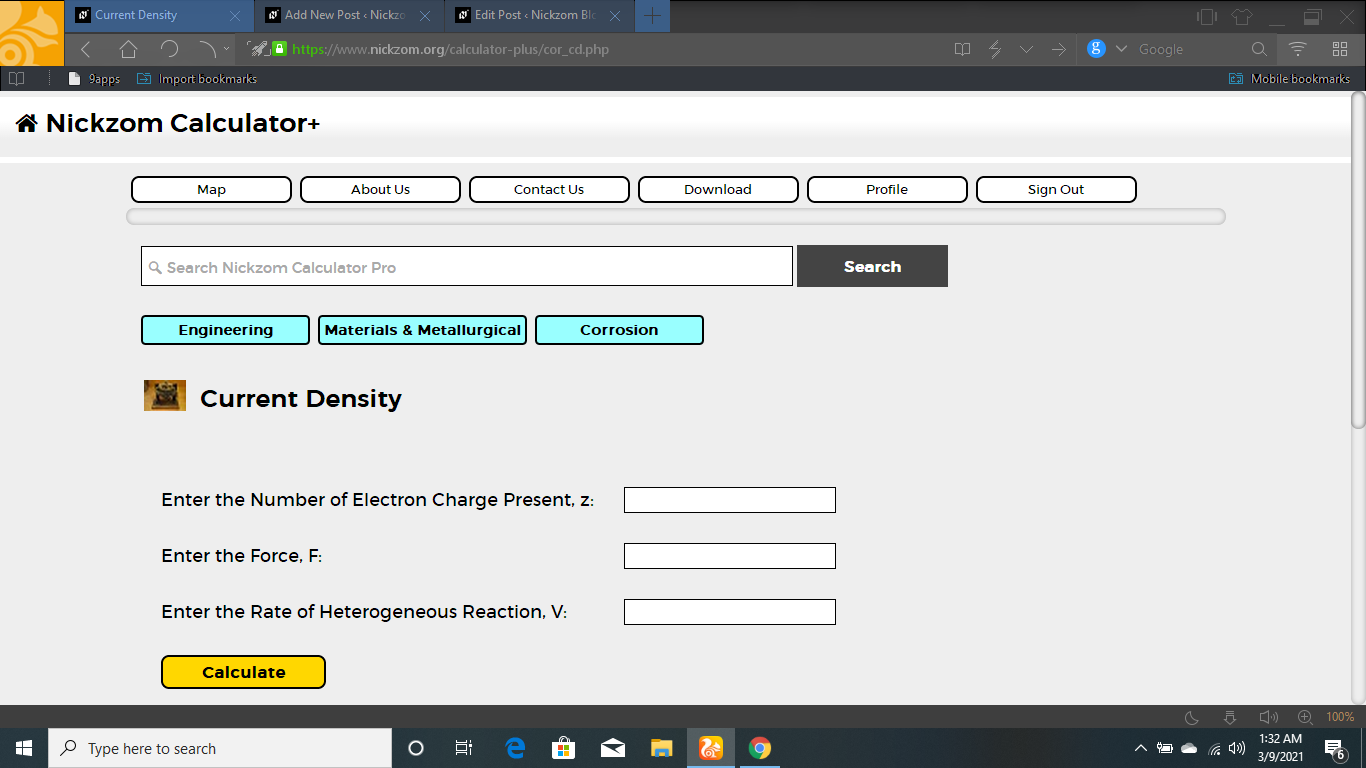Now, enter the value appropriately and accordingly for the parameter as required by the Number of Electron Charge Present (z) is 6, Force (F) is and Rate of Heterogeneous Reaction (V) is 8.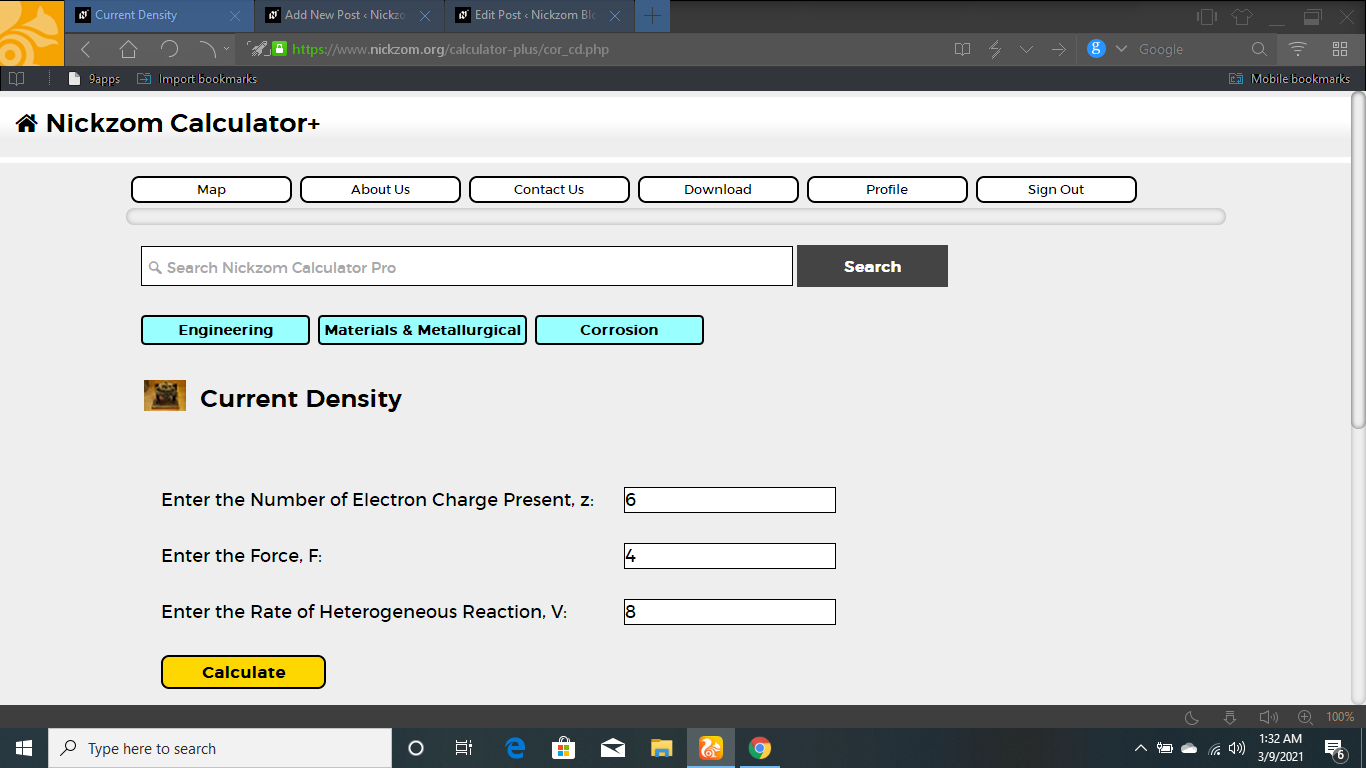Finally, Click on Calculate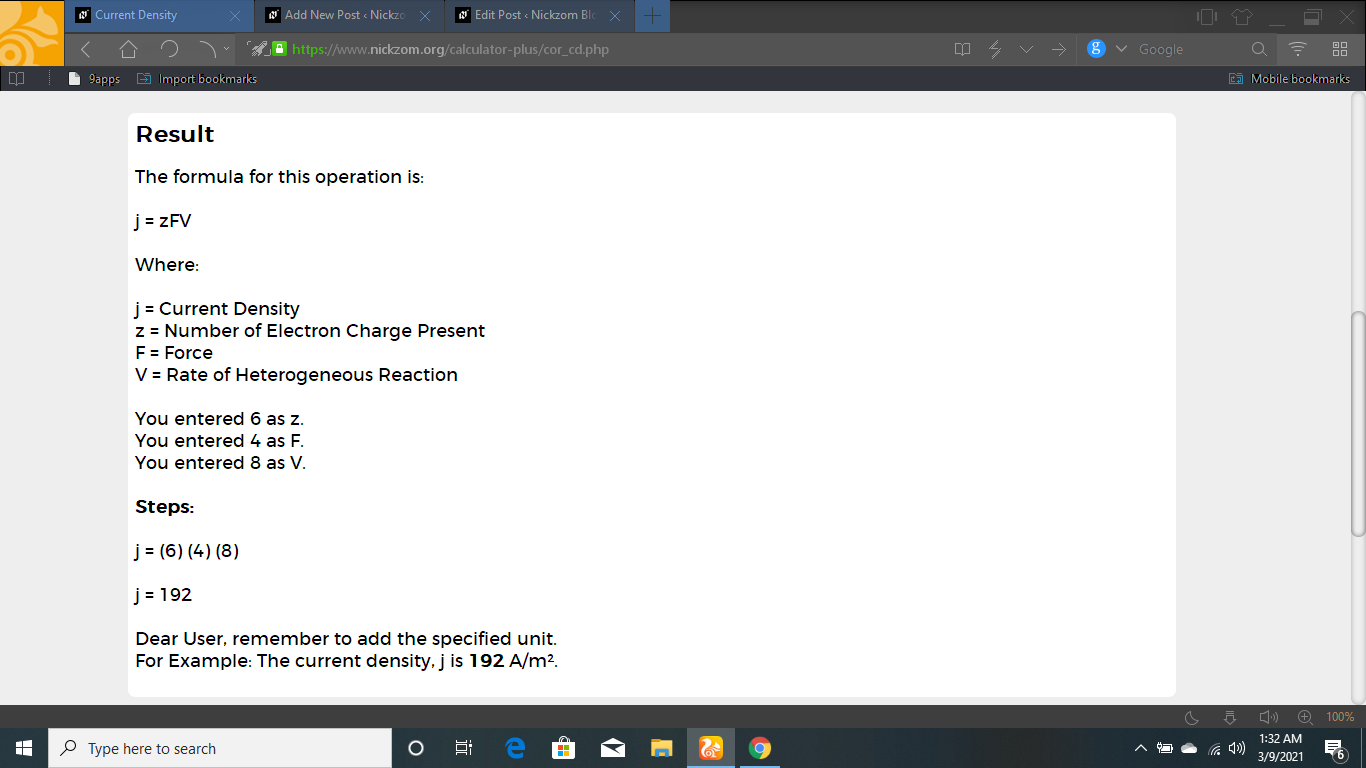As you can see from the screenshot above, Nickzom Calculator– The Calculator Encyclopedia solves for the current density and presents the formula, workings and steps too.